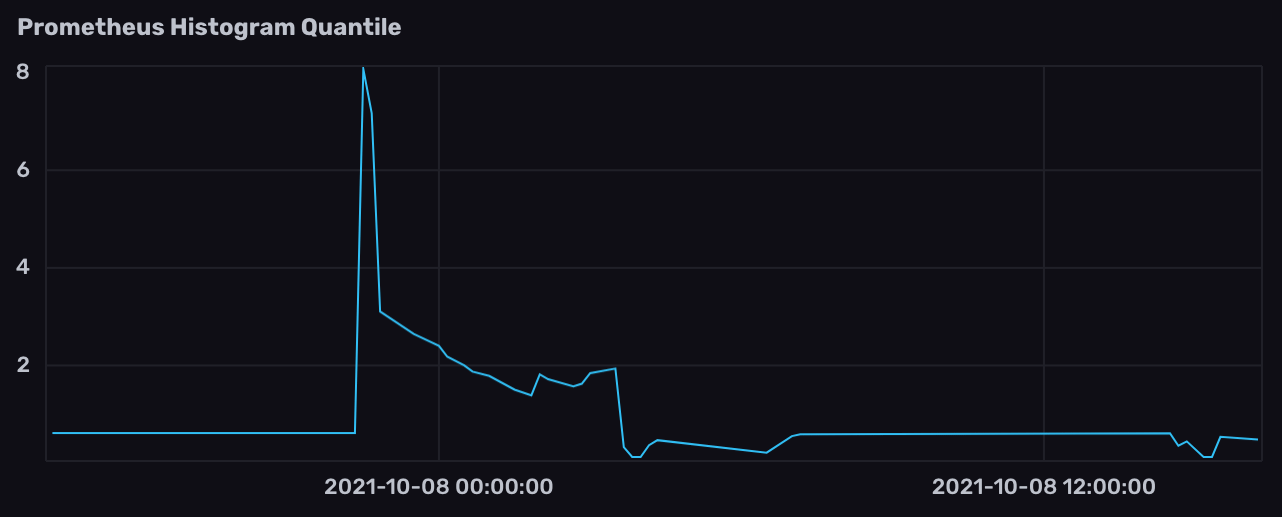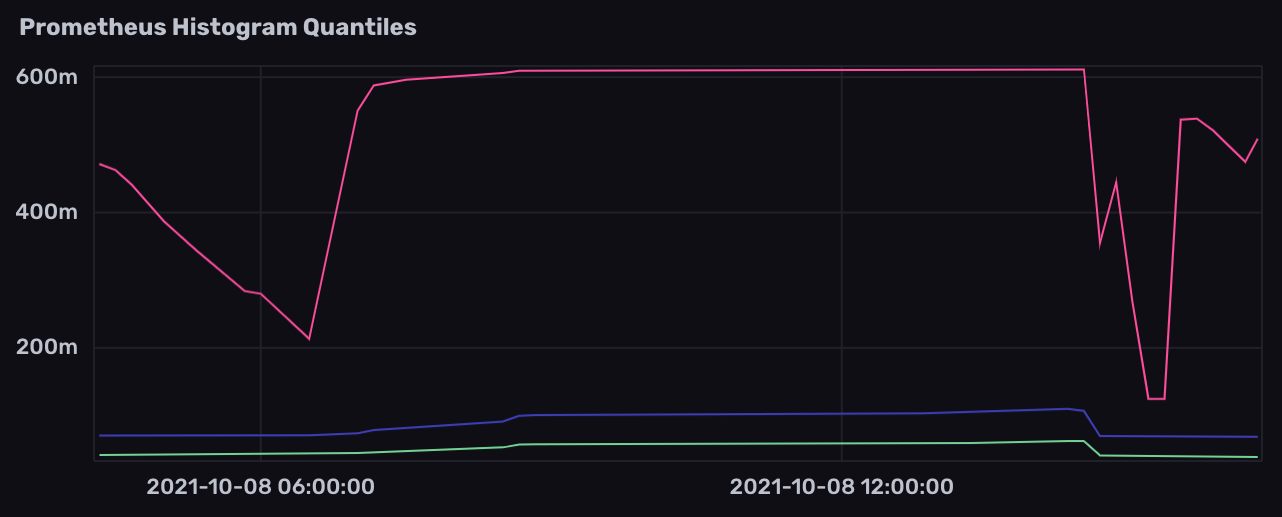# Work with Prometheus histograms

Use Flux to query and transform Prometheus histogram metrics stored in InfluxDB.

A histogram samples observations (usually things like request durations or response sizes) and counts them in configurable buckets. It also provides a sum of all observed values.

Prometheus metric types

##### Example histogram metric in Prometheus data
``````# HELP example_histogram_duration Duration of given tasks as example histogram metric
# TYPE example_histogram_duration histogram
example_histogram_duration_bucket{le="0.1"} 80
example_histogram_duration_bucket{le="0.25"} 85
example_histogram_duration_bucket{le="0.5"} 85
example_histogram_duration_bucket{le="1"} 87
example_histogram_duration_bucket{le="2.5"} 87
example_histogram_duration_bucket{le="5"} 88
example_histogram_duration_bucket{le="+Inf"} 88
example_histogram_duration_sum 6.833441910000001
example_histogram_duration_count 88
``````

The examples below include example data collected from the InfluxDB OSS 2.x `/metrics` endpoint and stored in InfluxDB.

#### Prometheus metric parsing formats

Query structure depends on the Prometheus metric parsing format used to scrape the Prometheus metrics. Select the appropriate metric format version below.

## Visualize Prometheus histograms in InfluxDB

InfluxDB does not currently support visualizing Prometheus histogram metrics as a traditional histogram. The existing InfluxDB histogram visualization is not compatible with the format of Prometheus histogram data stored in InfluxDB.

## Calculate quantile values from Prometheus histograms

1. Import the `experimental/prometheus` package.
2. Filter results by the `prometheus` measurement and histogram metric name field.
3. (Recommended) Use `aggregateWindow()` to downsample data and optimize the query.
4. Use `prometheus.histogramQuantile()` to calculate a specific quantile.
``````import "experimental/prometheus"

from(bucket: "example-bucket")
|> start(range: -1h)
|> filter(fn: (r) => r._measurement == "prometheus")
|> filter(fn: (r) => r._field == "qc_all_duration_seconds")
|> aggregateWindow(every: 1m, fn: mean, createEmpty: false)
|> prometheus.histogramQuantile(quantile: 0.99)
``````
1. Import the `experimental/prometheus` package.
2. Filter results by the histogram metric name measurement.
3. (Recommended) Use `aggregateWindow()` to downsample data and optimize the query. Set the `createEmpty` parameter to `false`.
4. Use `prometheus.histogramQuantile()` to calculate a specific quantile. Specify the `metricVersion` as `1`.
``````import "experimental/prometheus"

from(bucket: "example-bucket")
|> start(range: -1h)
|> filter(fn: (r) => r._measurement == "qc_all_duration_seconds")
|> aggregateWindow(every: 1m, fn: mean, createEmpty: false)
|> prometheus.histogramQuantile(quantile: 0.99, metricVersion: 1)
``````#### Set createEmpty to false

When using `aggregateWindow()` to downsample data for `prometheus.histogramQuantile`, set the `createEmpty` parameter to `false`. Empty tables produced from `aggregateWindow()` result in the following error.

``````histogramQuantile: unexpected null in the countColumn
``````

## Calculate multiple quantiles from Prometheus histograms

1. Query histogram data using steps 1-2 (optionally 3) from above.
2. Use `union()` to union multiple streams of tables that calculate unique quantiles.
``````import "experimental/prometheus"

data =
from(bucket: "example-bucket")
|> start(range: -1h)
|> filter(fn: (r) => r._measurement == "prometheus")
|> filter(fn: (r) => r._field == "qc_all_duration_seconds")
|> aggregateWindow(every: 1m, fn: mean, createEmpty: false)

union(
tables: [
data |> prometheus.histogramQuantile(quantile: 0.99),
data |> prometheus.histogramQuantile(quantile: 0.5),
data |> prometheus.histogramQuantile(quantile: 0.25),
],
)
``````
``````import "experimental/prometheus"

data =
from(bucket: "example-bucket")
|> start(range: -1h)
|> filter(fn: (r) => r._measurement == "qc_all_duration_seconds")
|> aggregateWindow(every: 1m, fn: mean, createEmpty: false)

union(
tables: [
data |> prometheus.histogramQuantile(quantile: 0.99, metricVersion: 1),
data |> prometheus.histogramQuantile(quantile: 0.5, metricVersion: 1),
data |> prometheus.histogramQuantile(quantile: 0.25, metricVersion: 1),
],
)
``````### Upgrade to InfluxDB Cloud or InfluxDB 2.0!

InfluxDB Cloud and InfluxDB OSS 2.0 ready for production.